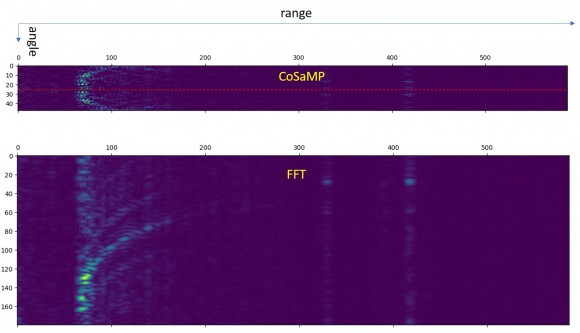## Compressed Sensing in DOA estimationStarted by 2 weeks ago91 views

I'm trying to apply the compressed sensing theory (CoSaMP algorithm) to the DOA estimation in FMCW ULA (made of 48 elements). In the dechirped signals processing I use a first FFT to solve the range and then instead to apply another FFT along the cross-range direction (following the standard 2D FFT) I use CoSaMP algorithm to get the direction of arrival bin by bin.

If we refer to the equation (per each single range bin): y = Φ Ψ s

y contains all the 48 FFT complex values, Φ is the measurement matrix that I use to select 30 out 48 antennas, Ψ is the representing FFT basis matrix, and s the sparse solution I look for (the same length of y vector)

In the below figure 1 the range-angle radar images that I got using a)CoSaMP (30 out 48 randomly chosen antennas) on the real part of the first (range) FFT complex output and b)FFT of order 180 on the first (range) FFT complex output. I have two main questions:

1. Is it possible to run the CoSaMP (or another CS algorithm) on complex values? As you can see in the image1 I got a symmetrical output due to the fact I'm applying the CoSaMP to the real values of the complex FFT output (range).
2. How I can improve the resolution in the sparse domain? This is subjected to the basis matrix dimension (in my case 48*48).

Thanks